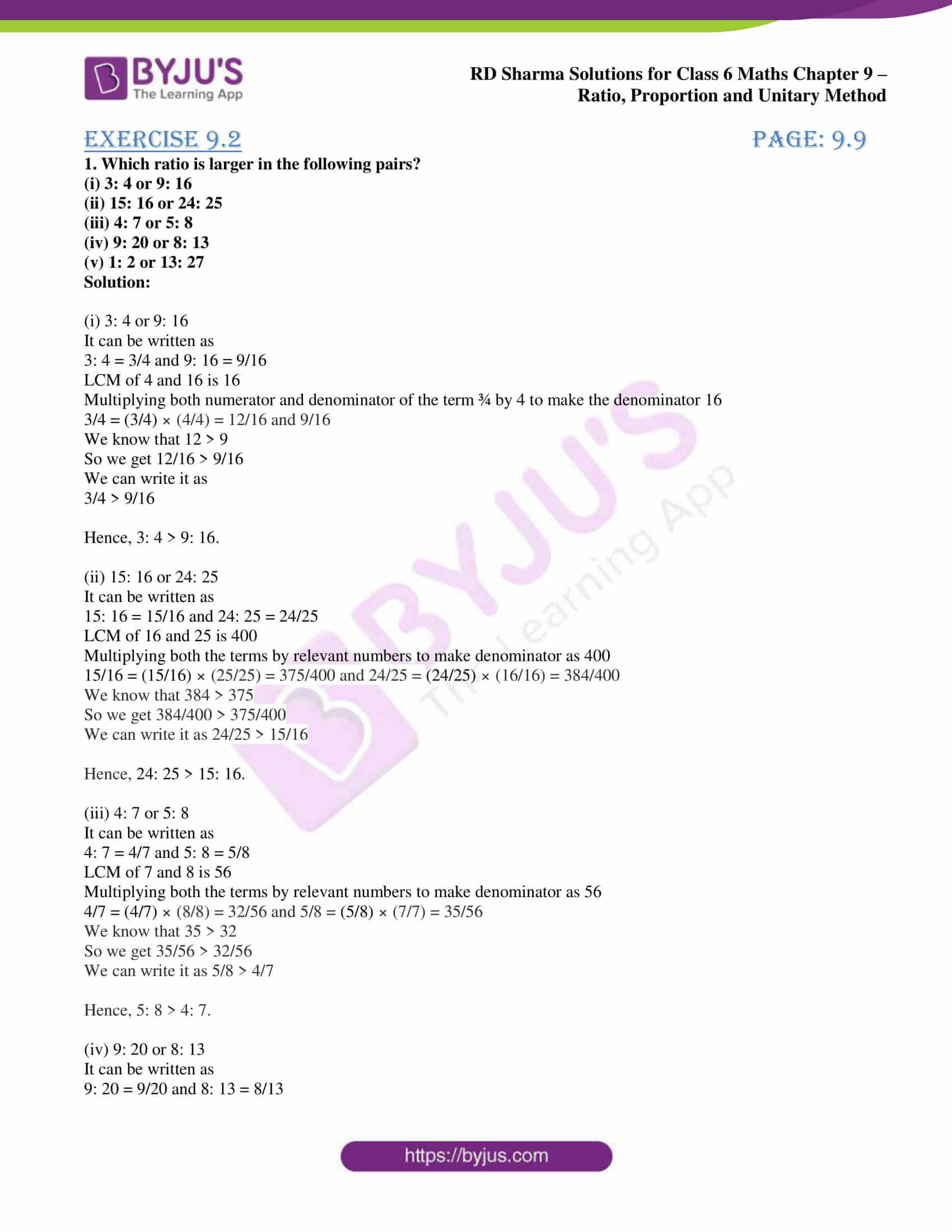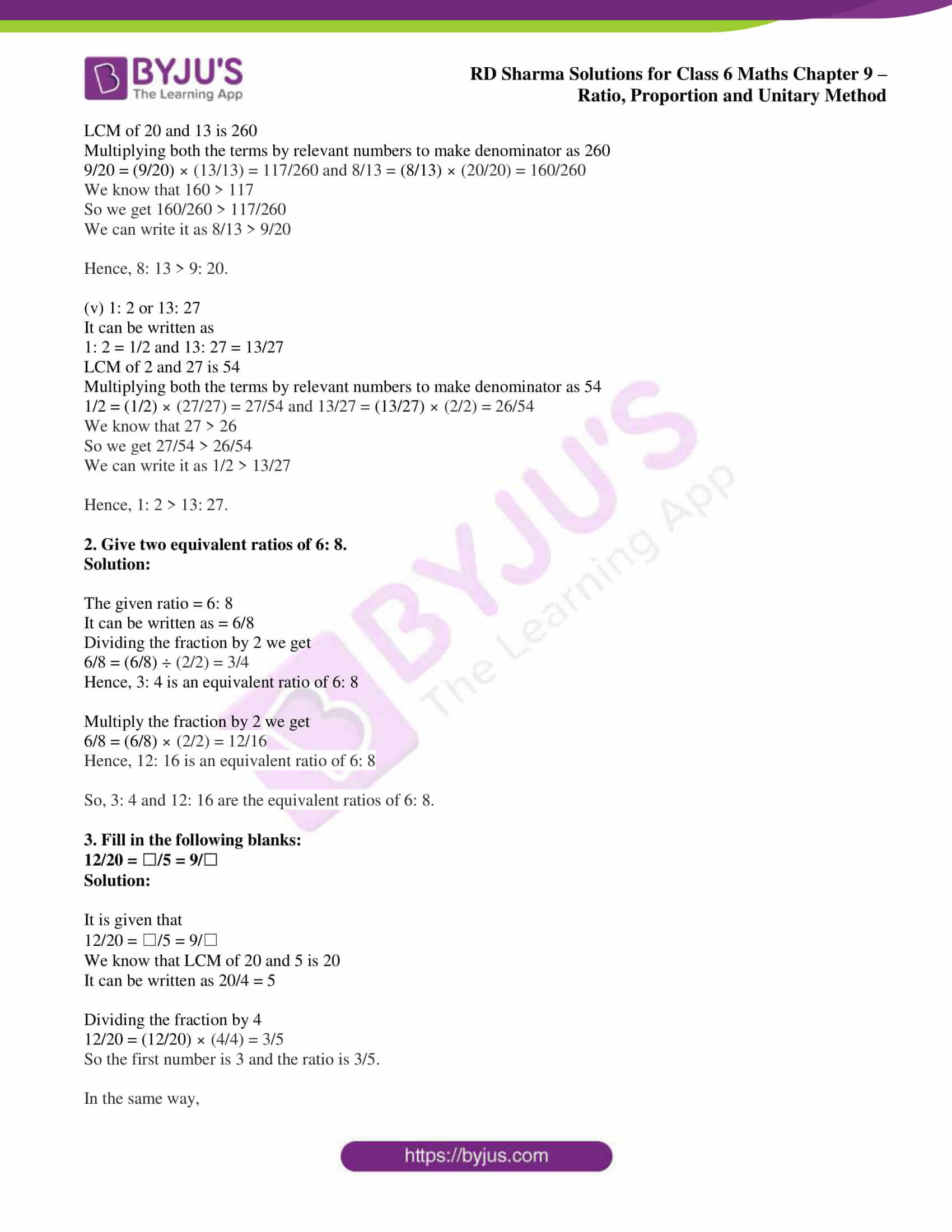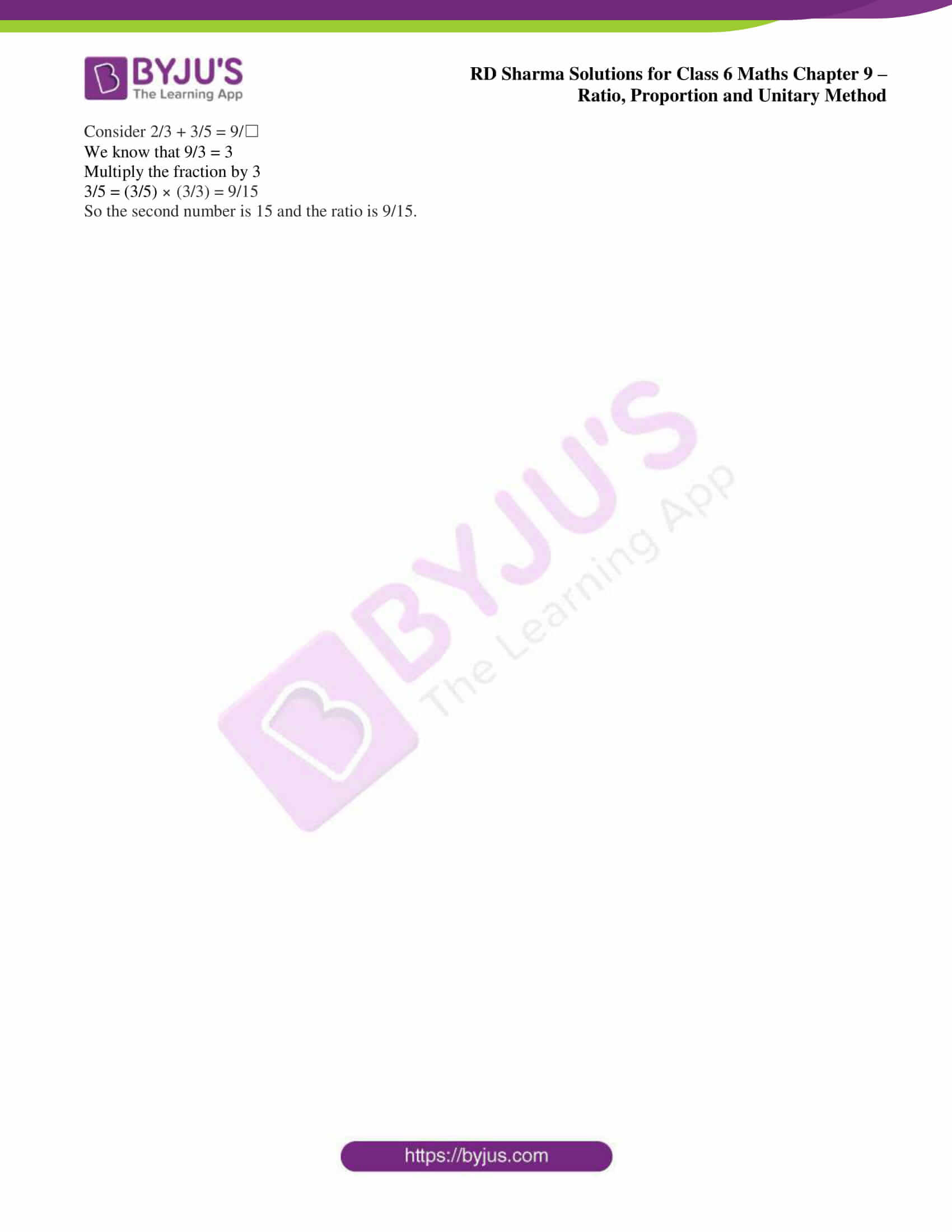# RD Sharma Solutions for Class 6 Maths Chapter 9: Ratio, Proportion and Unitary Method Exercise 9.2

This exercise helps students understand the steps which are followed in a comparison of ratios. RD Sharma Solutions Class 6 is a vital study material which helps students boost their speed in solving problems. The students can refer to the PDF while solving problems from RD Sharma textbook to clear doubts by themselves. To make learning easy for the students, RD Sharma Solutions for Class 6 Maths Chapter 9 Ratio, Proportion and Unitary Method Exercise 9.2 PDF are provided here.

## RD Sharma Solutions for Class 6 Maths Chapter 9: Ratio, Proportion and Unitary Method Exercise 9.2 Download PDF### Access answers to Maths RD Sharma Solutions for Class 6 Chapter 9: Ratio, Proportion and Unitary Method Exercise 9.2

(i) 3: 4 or 9: 16

(ii) 15: 16 or 24: 25

(iii) 4: 7 or 5: 8

(iv) 9: 20 or 8: 13

(v) 1: 2 or 13: 27

Solution:

(i) 3: 4 or 9: 16

It can be written as

3: 4 = 3/4 and 9: 16 = 9/16

LCM of 4 and 16 is 16

Multiplying both numerator and denominator of the term ¾ by 4 to make the denominator 16

3/4 = (3/4) × (4/4) = 12/16 and 9/16

We know that 12 > 9

So we get 12/16 > 9/16

We can write it as

3/4 > 9/16

Hence, 3: 4 > 9: 16.

(ii) 15: 16 or 24: 25

It can be written as

15: 16 = 15/16 and 24: 25 = 24/25

LCM of 16 and 25 is 400

Multiplying both the terms by relevant numbers to make denominator as 400

15/16 = (15/16) × (25/25) = 375/400 and 24/25 = (24/25) × (16/16) = 384/400

We know that 384 > 375

So we get 384/400 > 375/400

We can write it as 24/25 > 15/16

Hence, 24: 25 > 15: 16.

(iii) 4: 7 or 5: 8

It can be written as

4: 7 = 4/7 and 5: 8 = 5/8

LCM of 7 and 8 is 56

Multiplying both the terms by relevant numbers to make denominator as 56

4/7 = (4/7) × (8/8) = 32/56 and 5/8 = (5/8) × (7/7) = 35/56

We know that 35 > 32

So we get 35/56 > 32/56

We can write it as 5/8 > 4/7

Hence, 5: 8 > 4: 7.

(iv) 9: 20 or 8: 13

It can be written as

9: 20 = 9/20 and 8: 13 = 8/13

LCM of 20 and 13 is 260

Multiplying both the terms by relevant numbers to make denominator as 260

9/20 = (9/20) × (13/13) = 117/260 and 8/13 = (8/13) × (20/20) = 160/260

We know that 160 > 117

So we get 160/260 > 117/260

We can write it as 8/13 > 9/20

Hence, 8: 13 > 9: 20.

(v) 1: 2 or 13: 27

It can be written as

1: 2 = 1/2 and 13: 27 = 13/27

LCM of 2 and 27 is 54

Multiplying both the terms by relevant numbers to make denominator as 54

1/2 = (1/2) × (27/27) = 27/54 and 13/27 = (13/27) × (2/2) = 26/54

We know that 27 > 26

So we get 27/54 > 26/54

We can write it as 1/2 > 13/27

Hence, 1: 2 > 13: 27.

2. Give two equivalent ratios of 6: 8.

Solution:

The given ratio = 6: 8

It can be written as = 6/8

Dividing the fraction by 2 we get

6/8 = (6/8) ÷ (2/2) = 3/4

Hence, 3: 4 is an equivalent ratio of 6: 8

Multiply the fraction by 2 we get

6/8 = (6/8) × (2/2) = 12/16

Hence, 12: 16 is an equivalent ratio of 6: 8

So, 3: 4 and 12: 16 are the equivalent ratios of 6: 8.

3. Fill in the following blanks:

12/20 = ☐/5 = 9/☐

Solution:

It is given that

12/20 = ☐/5 = 9/☐

We know that LCM of 20 and 5 is 20

It can be written as 20/4 = 5

Dividing the fraction by 4

12/20 = (12/20) × (4/4) = 3/5

So the first number is 3 and the ratio is 3/5.

In the same way,

Consider 2/3 + 3/5 = 9/☐

We know that 9/3 = 3

Multiply the fraction by 3

3/5 = (3/5) × (3/3) = 9/15

So the second number is 15 and the ratio is 9/15.